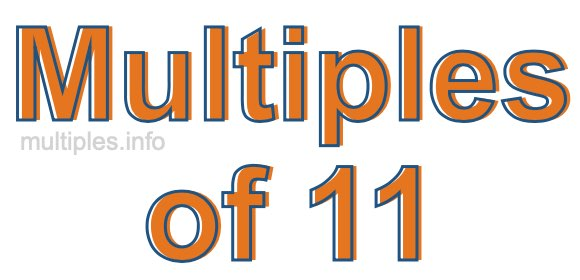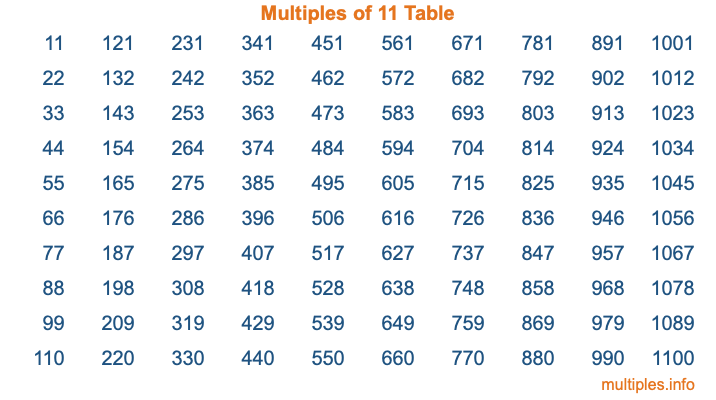Multiples of 11Welcome to the Multiples of 11 page. Here we will first teach you everything you will ever need to know about the multiples of 11, and then give you a study guide summary of everything we taught you to make sure you remember it all. Use this page to look up facts and learn information about the multiples of 11. This page will make you a multiples of eleven expert!

Definition of Multiples of 11
Multiples of 11 are all the numbers that when divided by 11 equal an integer. Each of the multiples of 11 are called a multiple. A multiple of 11 is created by multiplying 11 by an integer.

Therefore, to create a list of multiples of 11, you start with 1 multiplied by 11, then 2 multiplied by 11, then 3 multiplied by 11, and so on for as long as you want. Thus, the list of the first five multiples of 11 is 11, 22, 33, 44, and 55. To see a larger list of multiples of 11, see the printable image of Multiples of 11 further down on this page. We also have a category where you can choose any nth multiple of 11.

Multiples of 11 Checker
The Multiples of 11 Checker below checks to see if any number of your choice is a multiple of 11. In other words, it checks to see if there is any number (integer) that when multiplied by 11 will equal your number. To do that, we divide your number by 11. If the the quotient is an integer, then your number is a multiple of 11.

Is  a multiple of 11?

Least Common Multiple of 11 and ...
A Least Common Multiple (LCM) is the lowest multiple that two or more numbers have in common. This is also called the smallest common multiple or lowest common multiple and is useful to know when you are adding our subtracting fractions. Enter one or more numbers below (11 is already entered) to find the LCM.

Check out our LCM Calculator if you need more details about the Least Common Multiple or if you need the LCM for different numbers for adding and subtraction fractions.

nth Multiple of 11
As we stated above, 11 is the first multiple of 11, 22 is the second multiple of 11, 33 is the third multiple of 11, and so on. Enter a number below to find the nth multiple of 11.

th multiple of 11

Multiples of 11 vs Factors of 11
11 is a multiple of 11 and a factor of 11, but that is where the similarities end. All postive multiples of 11 are 11 or greater than 11. All positive factors of 11 are 11 or less than 11.

Below is the beginning list of multiples of 11 and the factors of 11 so you can compare:

Multiples of 11: 11, 22, 33, 44, 55, etc.

Factors of 11: 1, 11

As you can see, the multiples of 11 are all the numbers that you can divide by 11 to get a whole number. The factors of 11, on the other hand, are all the whole numbers that you can multiply by another whole number to get 11.

It's also interesting to note that if a number (x) is a factor of 11, then 11 will also be a multiple of that number (x).

Multiples of 11 vs Divisors of 11
The divisors of 11 are all the integers that 11 can be divided by evenly. Below is a list of the divisors of 11.

Divisors of 11: 1, 11

The interesting thing to note here is that if you take any multiple of 11 and divide it by a divisor of 11, you will see that the quotient is an integer.

Multiples of 11 Table
Below is an image of the first 100 multiples of 11 in a table. The table is in chronological order, column by column. The first column has the first ten multiples of 11, the second column has the next ten multiples of 11, and so on.The Multiples of 11 Table is also referred to as the 11 Times Table or Times Table of 11. You are welcome to print out our table for your studies.

Negative Multiples of 11
Although not often discussed or needed in math, it is worth mentioning that you can make a list of negative multiples of 11 by multiplying 11 by -1, then by -2, then by -3, and so on, to get the following list of negative multiples of 11:

-11, -22, -33, -44, -55, etc.

Multiples of 11 Summary
Below is a summary of important Multiples of 11 facts that we have discussed on this page. To retain the knowledge on this page, we recommend that you read through the summary and explain to yourself or a study partner why they hold true.

There are an infinite number of multiples of 11.

A multiple of 11 divided by 11 will equal a whole number.

11 divided by a factor of 11 equals a divisor of 11.

The nth multiple of 11 is n times 11.

The largest factor of 11 is equal to the first positive multiple of 11.

11 is a multiple of every factor of 11.

11 is a multiple of 11.

A multiple of 11 divided by a divisor of 11 equals an integer.

11 divided by a divisor of 11 equals a factor of 11.

Any integer times 11 will equal a multiple of 11.

Multiples of a Number
Here you can get the multiples of another number, all with the same attention to detail as we did for multiples of 11 on this page.

Multiples of
Multiples of 12
Did you find our page about multiples of eleven educational? Do you want more knowledge? Check out the multiples of the next number on our list!